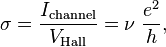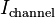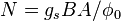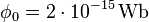# Quantum Hall effect

The quantum Hall effect (or integer quantum Hall effect) is a quantum-mechanical version of the Hall effect, observed in two-dimensional electron systems subjected to low temperatures and strong magnetic fields, in which the Hall conductance G undergoes certain quantum Hall transitions to take on the quantized values$sigma = frac{I_text{channel}}{V_text{Hall}} = nu ; frac{e^2}{h},$

where$I_text{channel}$ is the channel current,$V_text{Hall}$ is the Hall voltage, e is the elementary charge and h is Planck’s constant. The prefactor ν is known as the “filling factor”, and can take on either integer (ν = 1, 2, 3, .. ) or fractional (ν = 1/3, 2/5, 3/7, 2/3, 3/5, 1/5, 2/9, 3/13, 5/2, 12/5 …) values. The quantum Hall effect is referred to as the integer or fractional quantum Hall effect depending on whether ν is an integer or fraction respectively. The integer quantum Hall effect is very well understood, and can be simply explained in terms of single-particle orbitals of an electron in a magnetic field (see Landau quantization). The fractional quantum Hall effect is more complicated, as its existence relies fundamentally on electron–electron interactions. Although the microscopic origins of the fractional quantum Hall effect are unknown, there are several phenomenological approaches that provide accurate approximations. For example the effect can be thought of as an integer quantum Hall effect, not of electrons but of charge-flux composites known as composite fermions. In 1988, it was proposed that there was quantum Hall effect without Landau levels. This quantum Hall effect is referred to as the quantum anomalous Hall (QAH) effect. There is also a new concept of the quantum spin Hall effect which is an analogue of the quantum Hall effect, where spin currents flow instead of charge currents.

## Applications

The quantization of the Hall conductance has the important property of being incredibly precise. Actual measurements of the Hall conductance have been found to be integer or fractional multiples of e2/h to nearly one part in a billion. This phenomenon, referred to as “exact quantization”, has been shown to be a subtle manifestation of the principle of gauge invariance. It has allowed for the definition of a new practical standard for electrical resistance, based on the resistance quantum given by the von Klitzing constant RK = h/e2 = 25812.807557(18) Ω. This is named after Klaus von Klitzing, the discoverer of exact quantization. Since 1990, a fixed conventional value RK-90 is used in resistance calibrations worldwide. The quantum Hall effect also provides an extremely precise independent determination of the fine structure constant, a quantity of fundamental importance in quantum electrodynamics.

## History

The integer quantization of the Hall conductance was originally predicted by Ando, Matsumoto, and Uemura in 1975, on the basis of an approximate calculation which they themselves did not believe to be true. Several workers subsequently observed the effect in experiments carried out on the inversion layer of MOSFETs. It was only in 1980 that Klaus von Klitzing, working at the high magnetic field laboratory in Grenoble with silicon-based samples developed by Michael Pepper and Gerhard Dorda, made the unexpected discovery that the Hall conductivity was exactly quantized. For this finding, von Klitzing was awarded the 1985 Nobel Prize in Physics. The link between exact quantization and gauge invariance was subsequently found by Robert Laughlin. Most integer quantum Hall experiments are now performed on gallium arsenide heterostructures, although many other semiconductor materials can be used. In 2007, the integer quantum Hall effect was reported in graphene at temperatures as high as room temperature, and in the oxide ZnO-MgxZn1-xO.

## Integer quantum Hall effect – Landau levelsIn two dimensions, when classical electrons are subjected to a magnetic field they follow circular cyclotron orbits. When the system is treated quantum mechanically, these orbits are quantized. The energy levels of these quantized orbitals take on discrete values:$E_n = hbar omega_c (n+1/2)$, where ωc = eB/m is the cyclotron frequency. These orbitals are known as Landau levels, and at weak magnetic fields, their existence gives rise to many interesting “quantum oscillations” such as the Shubnikov–de Haas oscillations and the de Haas–van Alphen effect (which is often used to map the Fermi surface of metals). For strong magnetic fields, each Landau level is highly degenerate (i.e. there are many single particle states which have the same energy En). Specifically, for a sample of area A, in magnetic field B, the degeneracy of each Landau level is$N = g_s BA/phi_0$ (where gs represents a factor of 2 for spin degeneracy, and$phi_0 = 2 cdot 10^{-15},{rm Wb}$ is the magnetic flux quantum). For sufficiently strong B-fields, each Landau level may have so many states that all of the free electrons in the system sit in only a few Landau levels; it is in this regime where one observes the quantum Hall effect.

## Mathematics